# Homework 3. Bayesian Tomatoes¶

Due Thursday, October 17, 11:59pm

In this assignment, you'll be analyzing movie reviews from Rotten Tomatoes. This assignment will cover:

• Working with web APIs
• Making and interpreting predictions from a Bayesian perspective
• Using the Naive Bayes algorithm to predict whether a movie review is positive or negative
• Using cross validation to optimize models

Useful libraries for this assignment

In :
%matplotlib inline

import json

import requests
import pandas as pd
import numpy as np
import matplotlib.pyplot as plt

pd.set_option('display.width', 500)
pd.set_option('display.max_columns', 30)

# set some nicer defaults for matplotlib
from matplotlib import rcParams

#these colors come from colorbrewer2.org. Each is an RGB triplet
dark2_colors = [(0.10588235294117647, 0.6196078431372549, 0.4666666666666667),
(0.8509803921568627, 0.37254901960784315, 0.00784313725490196),
(0.4588235294117647, 0.4392156862745098, 0.7019607843137254),
(0.9058823529411765, 0.1607843137254902, 0.5411764705882353),
(0.4, 0.6509803921568628, 0.11764705882352941),
(0.9019607843137255, 0.6705882352941176, 0.00784313725490196),
(0.6509803921568628, 0.4627450980392157, 0.11372549019607843),
(0.4, 0.4, 0.4)]

rcParams['figure.figsize'] = (10, 6)
rcParams['figure.dpi'] = 150
rcParams['axes.color_cycle'] = dark2_colors
rcParams['lines.linewidth'] = 2
rcParams['axes.grid'] = False
rcParams['axes.facecolor'] = 'white'
rcParams['font.size'] = 14
rcParams['patch.edgecolor'] = 'none'

def remove_border(axes=None, top=False, right=False, left=True, bottom=True):
"""
Minimize chartjunk by stripping out unnecessary plot borders and axis ticks

The top/right/left/bottom keywords toggle whether the corresponding plot border is drawn
"""
ax = axes or plt.gca()
ax.spines['top'].set_visible(top)
ax.spines['right'].set_visible(right)
ax.spines['left'].set_visible(left)
ax.spines['bottom'].set_visible(bottom)

#turn off all ticks
ax.yaxis.set_ticks_position('none')
ax.xaxis.set_ticks_position('none')

#now re-enable visibles
if top:
ax.xaxis.tick_top()
if bottom:
ax.xaxis.tick_bottom()
if left:
ax.yaxis.tick_left()
if right:
ax.yaxis.tick_right()

In :
from ggplot import *


## Introduction¶

Rotten Tomatoes gathers movie reviews from critics. An entry on the website typically consists of a short quote, a link to the full review, and a Fresh/Rotten classification which summarizes whether the critic liked/disliked the movie.

When critics give quantitative ratings (say 3/4 stars, Thumbs up, etc.), determining the Fresh/Rotten classification is easy. However, publications like the New York Times don't assign numerical ratings to movies, and thus the Fresh/Rotten classification must be inferred from the text of the review itself.

This basic task of categorizing text has many applications. All of the following questions boil down to text classification:

• Is a movie review positive or negative?
• Is an email spam, or not?
• Is a comment on a blog discussion board appropriate, or not?

Language is incredibly nuanced, and there is an entire field of computer science dedicated to the topic (Natural Language Processing). Nevertheless, we can construct basic language models using fairly straightforward techniques.

## The Data¶

You will be starting with a database of Movies, derived from the MovieLens dataset. This dataset includes information for about 10,000 movies, including the IMDB id for each movie.

### Working with Web APIs¶

Web APIs are a more convenient way for programs to interact with websites. Rotten Tomatoes has a nice API that gives access to its data in JSON format.

To use this, you will first need to register for an API key. For "application URL", you can use anything -- it doesn't matter.

After you have a key, the documentation page shows the various data you can fetch from Rotten Tomatoes -- each type of data lives at a different web address. The basic pattern for fetching this data with Python is as follows (compare this to the Movie Reviews tab on the documentation page):

In :
api_key = 'jcquk524tumhccdenfy9pt7v'
movie_id = '770672122'  # toy story 3
url = 'http://api.rottentomatoes.com/api/public/v1.0/movies/%s/reviews.json' % movie_id

#these are "get parameters"
options = {'review_type': 'top_critic', 'page_limit': 20, 'page': 1, 'apikey': api_key}
data = requests.get(url, params=options).text
data = json.loads(data)  # load a json string into a collection of lists and dicts

print json.dumps(data['reviews'], indent=2)  # dump an object into a json string

{
"publication": "Village Voice",
"review": "http://www.villagevoice.com/2010-06-15/film/toys-are-us-in-toy-story-3/full/"
},
"quote": "When teenaged Andy plops down on the grass to share his old toys with a shy little girl, the film spikes with sadness and layered pleasure -- a concise, deeply wise expression of the ephemeral that feels real and yet utterly transporting.",
"freshness": "fresh",
"critic": "Eric Hynes",
"date": "2013-08-04"
}


## Part 1: Get the data¶

Here's a chunk of the MovieLens Dataset:

In :
from io import StringIO
movie_txt = requests.get('https://raw.github.com/cs109/cs109_data/master/movies.dat').text
movie_file = StringIO(movie_txt) # treat a string like a file

#print the first row
movies[['id', 'title', 'imdbID', 'year']].irow(0)

Out:
id                1
title     Toy story
imdbID       114709
year           1995
Name: 0, dtype: object

#### P1.1¶

We'd like you to write a function that looks up the first 20 Top Critic Rotten Tomatoes reviews for a movie in the movies dataframe. This involves two steps:

1. Use the Movie Alias API to look up the Rotten Tomatoes movie id from the IMDB id
2. Use the Movie Reviews API to fetch the first 20 top-critic reviews for this movie

Not all movies have Rotten Tomatoes IDs. In these cases, your function should return None. The detailed spec is below. We are giving you some freedom with how you implement this, but you'll probably want to break this task up into several small functions.

Hint In some situations, the leading 0s in front of IMDB ids are important. IMDB ids have 7 digits

In :
"""
Function
--------
fetch_reviews(movies, row)

Use the Rotten Tomatoes web API to fetch reviews for a particular movie

Parameters
----------
movies : DataFrame
The movies data above
row : int
The row of the movies DataFrame to use

Returns
-------
If you can match the IMDB id to a Rotten Tomatoes ID:
A DataFrame, containing the first 20 Top Critic reviews
for the movie. If a movie has less than 20 total reviews, return them all.
This should have the following columns:
critic : Name of the critic
fresh  : 'fresh' or 'rotten'
imdb   : IMDB id for the movie
publication: Publication that the critic writes for
quote  : string containing the movie review quote
review_data: Date of review
rtid   : Rotten Tomatoes ID for the movie
title  : Name of the movie

If you cannot match the IMDB id to a Rotten Tomatoes ID, return None

Examples
--------
>>> reviews = fetch_reviews(movies, 0)
>>> print len(reviews)
20
>>> print reviews.irow(1)
fresh                                                      fresh
imdb                                                      114709
publication                                             Time Out
quote          So ingenious in concept, design and execution ...
review_date                                           2009-10-04
rtid                                                        9559
title                                                  Toy story
Name: 1, dtype: object
"""

def get_movie_id(imdb_id):
url = 'http://api.rottentomatoes.com/api/public/v1.0/movie_alias.json'
imdb_id = '%.7i' % imdb_id
options = {'apikey': api_key, 'type': 'imdb', 'id': imdb_id}
r = requests.get(url, params=options)
if 'id' in r.json():
return r.json()['id'], r.json()['title']
else:
return None, None

def get_top_reviews(movie_id, n=20):
url = 'http://api.rottentomatoes.com/api/public/v1.0/movies/%i/reviews.json' % movie_id
options = {'apikey': api_key, 'page_limit': n, 'review_type': 'top_critic'}
r = requests.get(url, params=options)
return r.json()['reviews']

def fetch_reviews(movies, row, n=20):
imdb_id = movies.ix[row]['imdbID']
rt_id, title = get_movie_id(imdb_id)
if rt_id is not None:
reviews = get_top_reviews(rt_id, n=n)

df = pd.DataFrame(reviews)
df = df.rename(columns={'freshness': 'fresh'})
df['imdb'] = imdb_id
df['rt_id'] = rt_id
df['review_date'] = df['links'].apply(lambda x: x[u'review'] if 'review' in x else None)
df['title'] = title
return df

fetch_reviews(movies, 0).irow(1)

Out:
critic                                                  Derek Adams
date                                                     2009-10-04
fresh                                                         fresh
original_score                                                  5/5
publication                                                Time Out
quote             So ingenious in concept, design and execution ...
imdb                                                         114709
rt_id                                                          9559
review_date       http://www.timeout.com/film/reviews/87745/toy-...
title                                                     Toy Story
Name: 1, dtype: object

#### P1.2¶

Use the function you wrote to retrieve reviews for the first 3,000 movies in the movies dataframe.

##### Hints¶
• Rotten Tomatoes limits you to 10,000 API requests a day. Be careful about this limit! Test your code on smaller inputs before scaling. You are responsible if you hit the limit the day the assignment is due :)
• This will take a while to download. If you don't want to re-run this function every time you restart the notebook, you can save and re-load this data as a CSV file. However, please don't submit this file
In :
"""
Function
--------
build_table

Parameters
----------
movies : DataFrame
The movies data above
rows : int
The number of rows to extract reviews for

Returns
--------
A dataframe
The data obtained by repeatedly calling fetch_reviews on the first rows
of movies, discarding the Nones,
and concatenating the results into a single DataFrame
"""

def build_table(movies, rows):
ans = pd.DataFrame()
for i in range(rows):
new_df = fetch_reviews(movies, i)
ans = pd.concat((ans, new_df), ignore_index=True)
return ans

In :
#you can toggle which lines are commented, if you

# critics = build_table(movies, 3000)
# critics.to_csv('data/critics.csv', index=False)

In :
#for this assignment, let's drop rows with missing data
critics = critics[~critics.quote.isnull()]
critics = critics[critics.fresh != 'none']
critics = critics[critics.quote.str.len() > 0]


A quick sanity check that everything looks ok at this point

In :
assert len(critics) > 10000


## Part 2: Explore¶

Before delving into analysis, get a sense of what these data look like. Answer the following questions. Include your code!

2.1 How many reviews, critics, and movies are in this dataset?

In :
critics

Out:
<class 'pandas.core.frame.DataFrame'>
Int64Index: 15610 entries, 0 to 15609
Data columns (total 10 columns):
critic            14841  non-null values
date              15610  non-null values
fresh             15610  non-null values
imdb              15610  non-null values
original_score    7631  non-null values
publication       15610  non-null values
quote             15610  non-null values
review_date       13150  non-null values
rt_id             15610  non-null values
title             15610  non-null values
dtypes: int64(2), object(8)

In :
#your code here
print 'Reviews', len(critics)
print 'Critics', len(critics.critic.dropna().drop_duplicates())
print 'Moviews', len(critics.title.dropna().drop_duplicates())

Reviews 15610
Critics 621
Moviews 1931


2.2 What does the distribution of number of reviews per reviewer look like? Make a histogram

In :
#Your code here
critics.groupby(by='critic').critic.count().hist(bins=range(20))

Out:
<matplotlib.axes.AxesSubplot at 0x10ac65550>
/Users/danielfrg/anaconda/envs/harvard-ds/lib/python2.7/site-packages/matplotlib/font_manager.py:1236: UserWarning: findfont: Font family ['serif'] not found. Falling back to Bitstream Vera Sans
(prop.get_family(), self.defaultFamily[fontext]))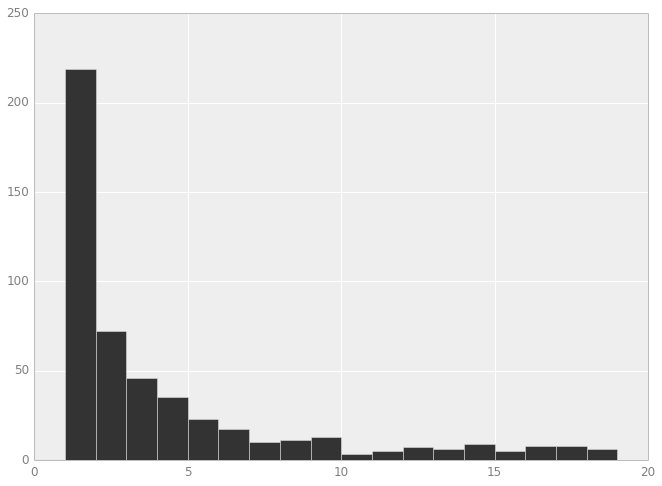2.3 List the 5 critics with the most reviews, along with the publication they write for

In :
#Your code here
top_critics = critics.groupby(by=['critic', 'publication']).critic.count()
top_critics.sort(ascending=False)

Out:
critic              publication
Roger Ebert         Chicago Sun-Times    1078
James Berardinelli  ReelViews             806
Janet Maslin        New York Times        519
Variety Staff       Variety               434
dtype: int64

2.4 Of the critics with > 100 reviews, plot the distribution of average "freshness" rating per critic

In :
#Your code here
critics['fresh_int'] = critics.fresh == 'fresh'
gb_critic = critics.groupby(by='critic')
n_reviews = gb_critic.critic.count()
means = gb_critic.fresh_int.mean()

means[n_reviews > 100].hist(bins=10)

Out:
<matplotlib.axes.AxesSubplot at 0x10199ef50>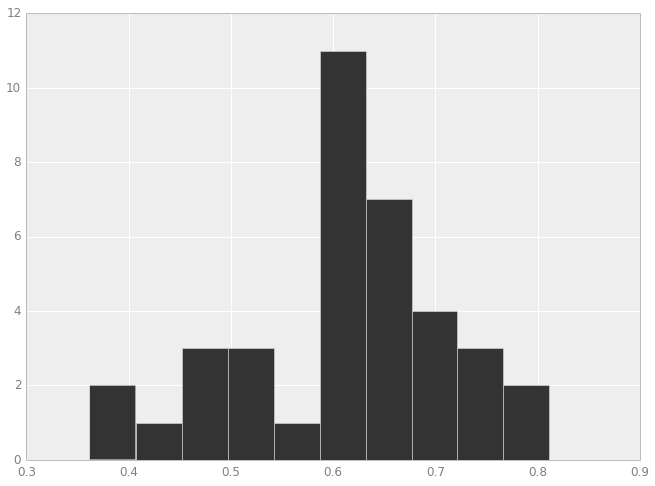2.5 Using the original movies dataframe, plot the rotten tomatoes Top Critics Rating as a function of year. Overplot the average for each year, ignoring the score=0 examples (some of these are missing data). Comment on the result -- is there a trend? What do you think it means?

In :
#Your code here
data = movies[['year', 'rtTopCriticsRating']]
data = data.convert_objects(convert_numeric=True)

ggplot(aes(x='year', y='rtTopCriticsRating'), data=data[data.rtTopCriticsRating > 0].sort('year')) + \
geom_point() + stat_smooth(color='red', se=True)

Out:
<ggplot: (278075917)>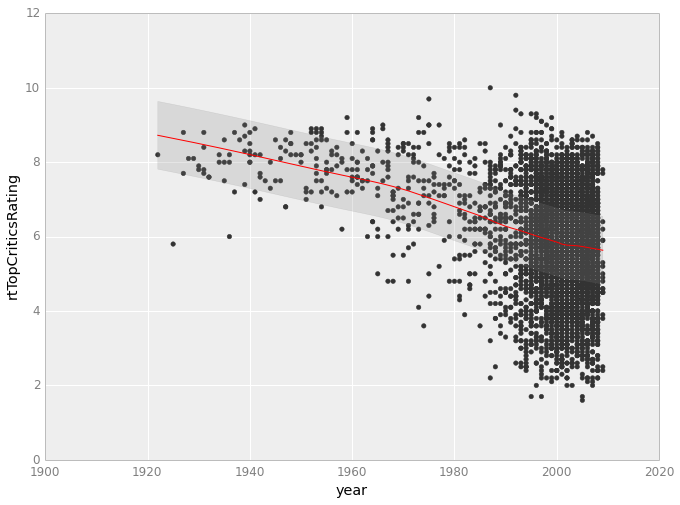New movies suck :P

## Part 3: Sentiment Analysis¶

You will now use a Naive Bayes classifier to build a prediction model for whether a review is fresh or rotten, depending on the text of the review. See Lecture 9 for a discussion of Naive Bayes.

Most models work with numerical data, so we need to convert the textual collection of reviews to something numerical. A common strategy for text classification is to represent each review as a "bag of words" vector -- a long vector of numbers encoding how many times a particular word appears in a blurb.

Scikit-learn has an object called a CountVectorizer that turns text into a bag of words. Here's a quick tutorial:

In :
from sklearn.feature_extraction.text import CountVectorizer

text = ['Hop on pop', 'Hop off pop', 'Hop Hop hop']
print "Original text is\n", '\n'.join(text)

vectorizer = CountVectorizer(min_df=0)

# call fit to build the vocabulary
vectorizer.fit(text)

# call transform to convert text to a bag of words
x = vectorizer.transform(text)

# CountVectorizer uses a sparse array to save memory, but it's easier in this assignment to
# convert back to a "normal" numpy array
x = x.toarray()

print
print "Transformed text vector is \n", x

# get_feature_names tracks which word is associated with each column of the transformed x
print
print "Words for each feature:"
print vectorizer.get_feature_names()

# Notice that the bag of words treatment doesn't preserve information about the *order* of words,
# just their frequency

Original text is
Hop on pop
Hop off pop
Hop Hop hop

Transformed text vector is
[[1 0 1 1]
[1 1 0 1]
[3 0 0 0]]

Words for each feature:
[u'hop', u'off', u'on', u'pop']


3.1

Using the critics dataframe, compute a pair of numerical X, Y arrays where:

• X is a (nreview, nwords) array. Each row corresponds to a bag-of-words representation for a single review. This will be the input to your model.
• Y is a nreview-element 1/0 array, encoding whether a review is Fresh (1) or Rotten (0). This is the desired output from your model.
In :
#hint: Consult the scikit-learn documentation to
#      learn about what these classes do do
from sklearn.cross_validation import train_test_split
from sklearn.naive_bayes import MultinomialNB

"""
Function
--------
make_xy

Build a bag-of-words training set for the review data

Parameters
-----------
critics : Pandas DataFrame
The review data from above

vectorizer : CountVectorizer object (optional)
A CountVectorizer object to use. If None,
then create and fit a new CountVectorizer.
Otherwise, re-fit the provided CountVectorizer
using the critics data

Returns
-------
X : numpy array (dims: nreview, nwords)
Bag-of-words representation for each review.
Y : numpy array (dims: nreview)
1/0 array. 1 = fresh review, 0 = rotten review

Examples
--------
X, Y = make_xy(critics)
"""
def make_xy(critics, vectorizer=None):
if vectorizer is None:
vectorizer = CountVectorizer()
X = vectorizer.fit_transform(critics.quote)
Y = (critics.fresh == 'fresh').values.astype(np.int)
return X, Y

In :
X, y = make_xy(critics)


3.2 Next, randomly split the data into two groups: a training set and a validation set.

Use the training set to train a MultinomialNB classifier, and print the accuracy of this model on the validation set

Hint You can use train_test_split to split up the training data

In :
#Your code here
from sklearn.cross_validation import train_test_split

X_train, X_test, y_train, y_test = train_test_split(X, y, test_size=0.3, random_state=1234)

In :
from sklearn.naive_bayes import MultinomialNB
clf = MultinomialNB()
clf.fit(X_train, y_train)

Out:
MultinomialNB(alpha=1.0, class_prior=None, fit_prior=True)
In :
clf.score(X_test, y_test)

Out:
0.77770659833440103

3.3:

We say a model is overfit if it performs better on the training data than on the test data. Is this model overfit? If so, how much more accurate is the model on the training data compared to the test data?

In :
# Your code here. Print the accuracy on the test and training dataset
clf.score(X_train, y_train)

Out:
0.9204722247643452

Interpret these numbers in a few sentences here

Yes, the model is overfitting because the accuracy on the trainig set is considerably higher than on the validation set.

3.4: Model Calibration

Bayesian models like the Naive Bayes classifier have the nice property that they compute probabilities of a particular classification -- the predict_proba and predict_log_proba methods of MultinomialNB compute these probabilities.

Being the respectable Bayesian that you are, you should always assess whether these probabilities are calibrated -- that is, whether a prediction made with a confidence of x% is correct approximately x% of the time. We care about calibration because it tells us whether we can trust the probabilities computed by a model. If we can trust model probabilities, we can make better decisions using them (for example, we can calculate how much we should bet or invest in a given prediction).

Let's make a plot to assess model calibration. Schematically, we want something like this:In words, we want to:

• Take a collection of examples, and compute the freshness probability for each using clf.predict_proba
• Gather examples into bins of similar freshness probability (the diagram shows 5 groups -- you should use something closer to 20)
• For each bin, count the number of examples in that bin, and compute the fraction of examples in the bin which are fresh
• In the upper plot, graph the expected P(Fresh) (x axis) and observed freshness fraction (Y axis). Estimate the uncertainty in observed freshness fraction $F$ via the equation $\sigma = \sqrt{F (1-F) / N}$
• Overplot the line y=x. This is the trend we would expect if the model is calibrated
• In the lower plot, show the number of examples in each bin

Hints

The output of clf.predict_proba(X) is a (N example, 2) array. The first column gives the probability $P(Y=0)$ or $P(Rotten)$, and the second gives $P(Y=1)$ or $P(Fresh)$.

The above image is just a guideline -- feel free to explore other options!

In :
"""
Function
--------
calibration_plot

Builds a plot like the one above, from a classifier and review data

Inputs
-------
clf : Classifier object
A MultinomialNB classifier
X : (Nexample, Nfeature) array
The bag-of-words data
Y : (Nexample) integer array
1 if a review is Fresh
"""

def calibration_plot(clf, X, y):
proba = clf.predict_proba(X)[:, 1]
data = pd.DataFrame({'proba': proba, 'fresh': y})

bins = np.linspace(0, 1, 20)
bin_center = (bins[:-1] + bins[1:]) / 2
cuts = pd.cut(proba, bins)

calibration = data.groupby(cuts).fresh.agg(['mean', 'count'])
calibration['bin_center'] = bin_center
calibration['std'] = np.sqrt(calibration.bin_center * (1 - calibration.bin_center) / calibration['count'])

print ggplot(aes(x='bin_center', y='mean'), data=calibration) + \
geom_point() + xlim(0, 1) + ylim(0, 1) + stat_smooth(color='red', se=True, span=0.25)

print ggplot(aes(x='proba'), data=data) + geom_histogram(binwidth=0.02) + xlim(0, 1)

In :
calibration_plot(clf, X_test, y_test)

<ggplot: (268715921)>
<ggplot: (280705005)>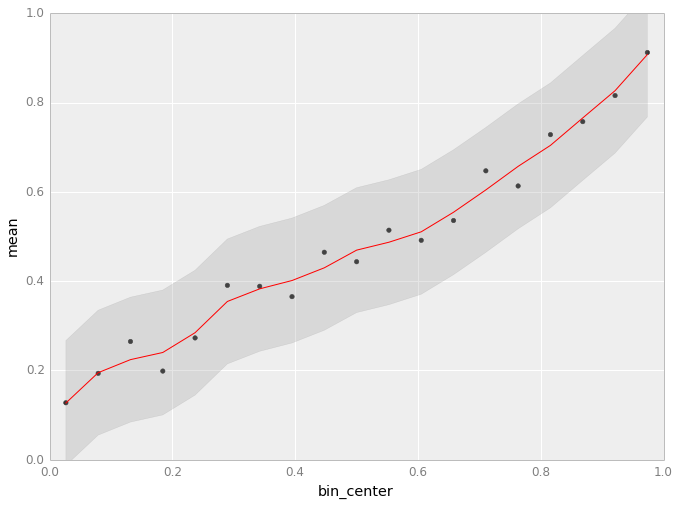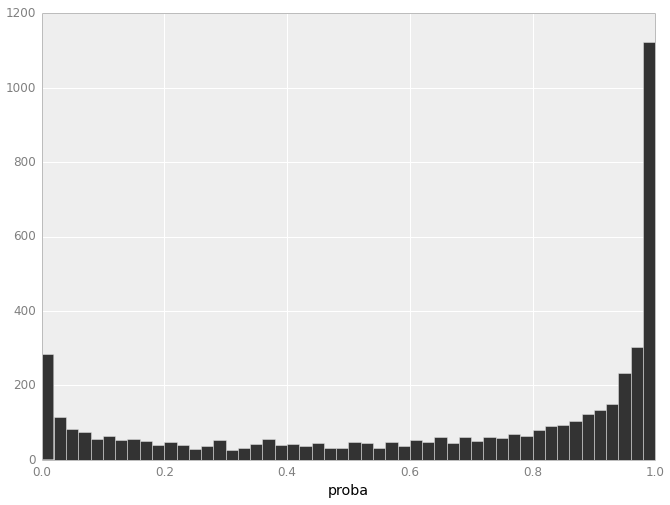3.5 We might say a model is over-confident if the freshness fraction is usually closer to 0.5 than expected (that is, there is more uncertainty than the model predicted). Likewise, a model is under-confident if the probabilities are usually further away from 0.5. Is this model generally over- or under-confident?

#### Cross Validation¶

Our classifier has a few free parameters. The two most important are:

1. The min_df keyword in CountVectorizer, which will ignore words which appear in fewer than min_df fraction of reviews. Words that appear only once or twice can lead to overfitting, since words which occur only a few times might correlate very well with Fresh/Rotten reviews by chance in the training dataset.

2. The alpha keyword in the Bayesian classifier is a "smoothing parameter" -- increasing the value decreases the sensitivity to any single feature, and tends to pull prediction probabilities closer to 50%.

As discussed in lecture and HW2, a common technique for choosing appropriate values for these parameters is cross-validation. Let's choose good parameters by maximizing the cross-validated log-likelihood.

3.6 Using clf.predict_log_proba, write a function that computes the log-likelihood of a dataset

In :
"""
Function
--------
log_likelihood

Compute the log likelihood of a dataset according to a bayesian classifier.
The Log Likelihood is defined by

L = Sum_fresh(logP(fresh)) + Sum_rotten(logP(rotten))

Where Sum_fresh indicates a sum over all fresh reviews,
and Sum_rotten indicates a sum over rotten reviews

Parameters
----------
clf : Bayesian classifier
x : (nexample, nfeature) array
The input data
y : (nexample) integer array
Whether each review is Fresh
"""

def log_likelihood(clf, x, y):
prob = clf.predict_log_proba(x)
fresh = y == 1
return prob[fresh, 1].sum() + prob[~fresh, 0].sum()

In :
log_likelihood(clf, X_test, y_test)

Out:
-2582.9773078870385

Here's a function to estimate the cross-validated value of a scoring function, given a classifier and data

In :
from sklearn.cross_validation import KFold

def cv_score(clf, x, y, score_func):
"""
Uses 5-fold cross validation to estimate a score of a classifier

Inputs
------
clf : Classifier object
x : Input feature vector
y : Input class labels
score_func : Function like log_likelihood, that takes (clf, x, y) as input,
and returns a score

Returns
-------
The average score obtained by randomly splitting (x, y) into training and
test sets, fitting on the training set, and evaluating score_func on the test set

Examples
cv_score(clf, x, y, log_likelihood)
"""
result = 0
nfold = 5
for train, test in KFold(y.size, nfold): # split data into train/test groups, 5 times
clf.fit(x[train], y[train]) # fit
result += score_func(clf, x[test], y[test]) # evaluate score function on held-out data
return result / nfold # average

# as a side note, this function is builtin to the newest version of sklearn. We could just write
# sklearn.cross_validation.cross_val_score(clf, x, y, scorer=log_likelihood).


3.7

Fill in the remaining code in this block, to loop over many values of alpha and min_df to determine which settings are "best" in the sense of maximizing the cross-validated log-likelihood

In :
#the grid of parameters to search over
alphas = [0, .1, 1, 5, 10, 50]
min_dfs = [1e-5, 1e-4, 1e-3, 1e-2, 1e-1]

#Find the best value for alpha and min_df, and the best classifier
best_alpha = None
best_min_df = None
max_loglike = -np.inf

for alpha in alphas:
for min_df in min_dfs:
vectorizer = CountVectorizer(min_df = min_df)
X, Y = make_xy(critics, vectorizer)

clf = MultinomialNB(alpha=alpha)
loglike = cv_score(clf, X, Y, log_likelihood)

if loglike > max_loglike:
max_loglike = loglike
best_alpha, best_min_df = alpha, min_df

In :
print "alpha: %f" % best_alpha
print "min_df: %f" % best_min_df

alpha: 5.000000
min_df: 0.001000


3.8 Now that you've determined values for alpha and min_df that optimize the cross-validated log-likelihood, repeat the steps in 3.1, 3.2, and 3.4 to train a final classifier with these parameters, re-evaluate the accuracy, and draw a new calibration plot.

In :
#Your code here

vectorizer = CountVectorizer(min_df=best_min_df)
X, y = make_xy(critics, vectorizer)
X_train, X_test, y_train, y_test = train_test_split(X, y, test_size=0.3, random_state=1234)

clf = MultinomialNB(alpha=best_alpha).fit(X_train, y_train)

calibration_plot(clf, X_test, y_test)

training_accuracy = clf.score(X_train, y_train)
test_accuracy = clf.score(X_test, y_test)

training_accuracy, test_accuracy

<ggplot: (280707197)>
<ggplot: (278472225)>

Out:
(0.78255696897593119, 0.74781123211616485)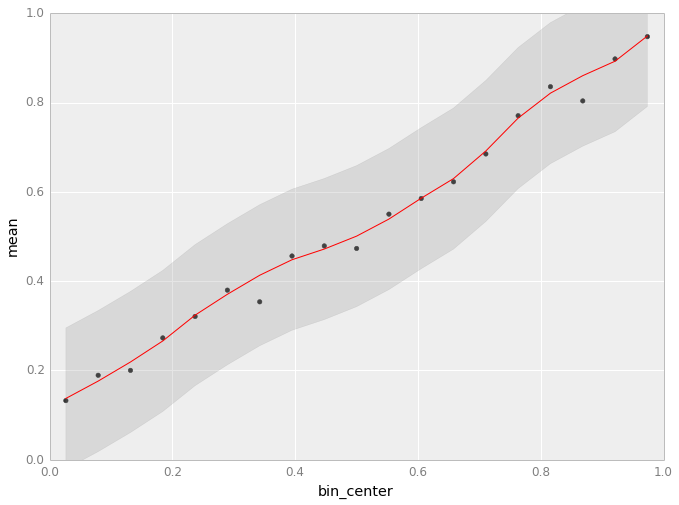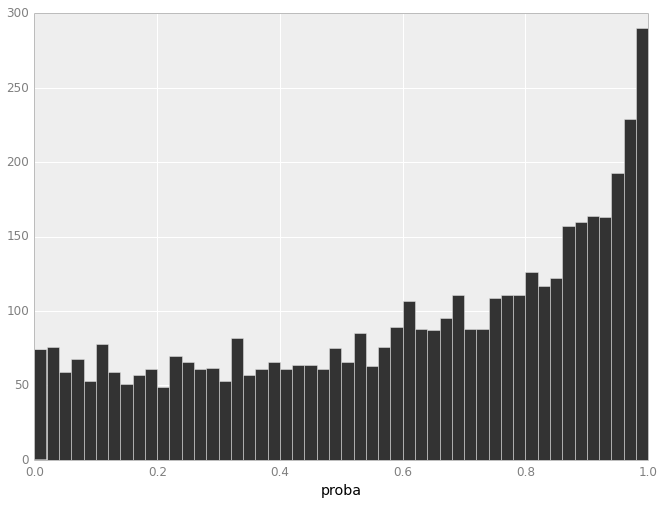3.9 Discuss the various ways in which Cross-Validation has affected the model. Is the new model more or less accurate? Is overfitting better or worse? Is the model more or less calibrated?

The grid search using crossvalidation gave better parameters and the overfitting was reduced a lot.

The calibration is also better.

To think about/play with, but not to hand in: What would happen if you tried this again using a function besides the log-likelihood -- for example, the classification accuracy?

## Part 4: Interpretation. What words best predict a fresh or rotten review?¶

4.1 Using your classifier and the vectorizer.get_feature_names method, determine which words best predict a positive or negative review. Print the 10 words that best predict a "fresh" review, and the 10 words that best predict a "rotten" review. For each word, what is the model's probability of freshness if the word appears one time?

#### Hints¶

• Try computing the classification probability for a feature vector which consists of all 0s, except for a single 1. What does this probability refer to?

• np.eye generates a matrix where the ith row is all 0s, except for the ith column which is 1.

In :
# Your code here

all_words = np.array(vectorizer.get_feature_names())

x = np.eye(X_test.shape)
probas = clf.predict_log_proba(x)[:, 0]
idx = np.argsort(probas)

good_words = pd.Series(1 - np.exp(probas[idx[:10]]), index=all_words[idx[:10]])
bad_words = pd.Series(1 - np.exp(probas[idx[-10:]]), index=all_words[idx[-10:]])

In :
good_words

Out:
delight         0.902645
masterpiece     0.881520
touching        0.873689
entertaining    0.866424
finest          0.863998
witty           0.860746
remarkable      0.857335
superb          0.849986
moving          0.848029
captures        0.847631
dtype: float64
In :
bad_words

Out:
dull              0.229352
tedious           0.227405
disappointment    0.227405
unfunny           0.227405
problem           0.215512
bland             0.199145
uninspired        0.189715
pointless         0.170839
unfortunately     0.163110
lame              0.142484
dtype: float64

4.2

One of the best sources for inspiration when trying to improve a model is to look at examples where the model performs poorly.

Find 5 fresh and rotten reviews where your model performs particularly poorly. Print each review.

In :
#Your code here
X, y = make_xy(critics, vectorizer)

probas = clf.predict_proba(X)[:, 1]
predict = clf.predict(X)

In :
bad_fresh = np.argsort(prob)[(y == 1) & (predict == 0)]

In :
data = critics[['title', 'fresh', 'quote']]

In :
data['prediction'] = predict
data['probas'] = probas

In :
bad_fresh = data.sort('probas')[(data.fresh == 'fresh') & (data.prediction == 0)]

In :
for index, row in bad_fresh[:5].iterrows():
print "Movie: %s | Correct: %s | Prediction: %i(%f)" % (row['title'], row['fresh'], row['prediction'], row['probas'])
print row['quote']
print

Movie: Young Frankenstein | Correct: fresh | Prediction: 0(0.001063)
Some of the gags don't work, but fewer than in any previous Brooks film that I've seen, and when the jokes are meant to be bad, they are riotously poor. What more can one ask of Mel Brooks?

Movie: The Fugitive | Correct: fresh | Prediction: 0(0.001567)
Though it's a good half hour too long, this overblown 1993 spin-off of the 60s TV show otherwise adds up to a pretty good suspense thriller.

Movie: Little Big Man | Correct: fresh | Prediction: 0(0.002598)
Might it be a serious attempt to right some unretrievable wrong via gallows humor which avoids the polemics? This seems to be the course taken; the attempt at least can be respected in theory.

Movie: Charlotte's Web | Correct: fresh | Prediction: 0(0.003695)
There's too much talent and too strong a story to mess it up. There was potential for more here, but this incarnation is nothing to be ashamed of, and some of the actors answer the bell.

Movie: Bad Boys | Correct: fresh | Prediction: 0(0.009323)
A good half-hour's worth of nonsense in the middle keeps Bad Boys from being little better than a break- even proposition.


In :
bad_rotten = data.sort('probas')[(data.fresh == 'rotten') & (data.prediction == 1)]

In :
for index, row in bad_rotten[:5].iterrows():
print "Movie: %s | Correct: %s | Prediction: %i(%f)" % (row['title'], row['fresh'], row['prediction'], row['probas'])
print row['quote']
print

Movie: Valkyrie | Correct: rotten | Prediction: 1(0.500475)
What you miss in both Defiance and Valkyrie is inner conflict. Their protagonists have not an instant of self-doubt. They're figures in historical pageants, not characters in a drama.

Movie: The Adventures of Buckaroo Banzai Across the 8th Dimension | Correct: rotten | Prediction: 1(0.500613)
It violates every rule of storytelling and narrative structure in creating a self-contained world of its own.

Movie: The Horse Whisperer | Correct: rotten | Prediction: 1(0.500899)
This has loads of craft and honor but never quite takes off.

Movie: Home for the Holidays | Correct: rotten | Prediction: 1(0.501135)
With many of the conversations going on simultaneously, it's difficult -- sometimes even impossible -- to know who is saying what and to whom.

Movie: Star Wars: Episode VI - Return of the Jedi | Correct: rotten | Prediction: 1(0.501448)
Let's not pretend we're watching art!



4.3 What do you notice about these mis-predictions? Naive Bayes classifiers assume that every word affects the probability independently of other words. In what way is this a bad assumption? In your answer, report your classifier's Freshness probability for the review "This movie is not remarkable, touching, or superb in any way".

In :
clf.predict_proba(vectorizer.transform(['This movie is not remarkable, touching, or superb in any way']))

Out:
array([[ 0.01948186,  0.98051814]])

Words like 'but' are used to negate the next words but the classifier does not pick those the negation only single words. The previous example shows this.

4.4 If this was your final project, what are 3 things you would try in order to build a more effective review classifier? What other exploratory or explanatory visualizations do you think might be helpful?

Nice visualization: A tag cloud with the tod words to predict fress vs rotten.

Improvements:

• Use N-grams to take into account interaction between words.
• Use more features besides only the quote.

### How to Submit¶

Restart and run your notebook one last time, to make sure the output from each cell is up to date. To submit your homework, create a folder named lastname_firstinitial_hw3 and place your solutions in the folder. Double check that the file is still called HW3.ipynb, and that it contains your code. Please do not include the critics.csv data file, if you created one. Compress the folder (please use .zip compression) and submit to the CS109 dropbox in the appropriate folder. If we cannot access your work because these directions are not followed correctly, we will not grade your work!

css tweaks in this cell